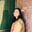Related Tags

c

# What are expressions in C?Umme Ammara

An expression in C is a combination of operands and operators – it computes a single value stored in a variable. The operator denotes the action or operation to be performed. The operands are the items to which we apply the operation.

Expression

An expression can be defined depending on the position and number of its operator and operands:

1. Infix Expression (operator is used between the operands)
a = x + y

1. Postfix Expression (operator is used after the operands)
xy+

1. Prefix Expression (operator is used before the operands)
+xy

1. Unary Expression (one operator and one operand)
x++

1. Binary Expression (one operator and two operands)
x+y


## Types of Expression

There are six types of expressions. These are shown below:

### Arithmetic Expression

It consists of arithmetic operators ( + , - , * , and / ) and computes values of int, float, or double type.

### Relational Expression

It usually consists of comparison operators (> , < , >= , <= , === , and !== ) and computes the answer in the bool type, i.e., true (1) or false (0).

### Logical Expression

It consists of logical operators (&&, ||, and !) and combines relational expressions to compute answers in the bool type.

### Conditional Expression

It consists of conditional statements that return true if the condition is met and false otherwise.

### Pointer Expression

It may consist of an ampersand (&) operator and returns address values.

### Bitwise Expression

It consists of bitwise operators ( >>, <<, ~, &, |, and ^ ) and performs operations at the bit level.

## Example

#include <stdio.h>

int main(){

//Arithmetic Expression
int a = (6 * 2) + 7 - 9;
printf("The arithmetic expression returns: %d\n", a);

//Relational Expression
int b = 10;
printf("The relational expression returns: %d\n", b % 2 == 0);

//Logical Expression
int c = (7 > 9) && ( 5 <= 9);
printf("The logical expression returns: %d\n", c);

//Conditional Expression
int d = (34 > 7) ? 1 : 0;
printf("The conditional expression returns: %d\n", d);

//Pointer Expression
int e = 20;
printf("The pointer expression returns: %p\n", addr);

//Bitwise Expression
int f = 10;
int shift = 10 >> 1;
printf("The bitwise expression returns: %d\n", shift);

return 0;
}

RELATED TAGS

c

CONTRIBUTORUmme Ammara